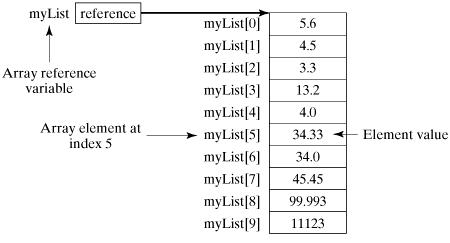# Array Declarations in Java

Object Oriented ProgrammingJava 8Programming

Here is the syntax for declaring an array variable −

## Syntax

dataType[] arrayRefVar;   // preferred way.
or
dataType arrayRefVar[];  // works but not preferred way.

Note − The style dataType[] arrayRefVar is preferred. The style dataType arrayRefVar[] comes from the C/C++ language and was adopted in Java to accommodate C/C++ programmers.

## Example

The following code snippets are examples of this syntax −

double[] myList;   // preferred way.
or
double myList[];   // works but not preferred way.

## Creating Arrays

You can create an array by using the new operator with the following syntax −

## Syntax

arrayRefVar = new dataType[arraySize];

The above statement does two things −

• It creates an array using new dataType[arraySize].

• It assigns the reference of the newly created array to the variable arrayRefVar.

Declaring an array variable, creating an array, and assigning the reference of the array to the variable can be combined in one statement, as shown below −

dataType[] arrayRefVar = new dataType[arraySize];

Alternatively, you can create arrays as follows −

dataType[] arrayRefVar = {value0, value1, ..., valuek};

The array elements are accessed through the index. Array indices are 0-based; that is, they start from 0 to arrayRefVar.length-1.

## Example

Following statement declares an array variable, myList, creates an array of 10 elements of double type and assigns its reference to myList −

double[] myList = new double;

The following picture represents array myList. Here, myList holds ten double values and the indices are from 0 to 9.## Processing Arrays

When processing array elements, we often use either for loop or foreach loop because all of the elements in an array are of the same type and the size of the array is known.

## Example

Here is a complete example showing how to create, initialize, and process arrays −

Live Demo

public class TestArray {

public static void main(String[] args) {
double[] myList = {1.9, 2.9, 3.4, 3.5};

// Print all the array elements
for (int i = 0; i < myList.length; i++) {
System.out.println(myList[i] + " ");
}

// Summing all elements
double total = 0;
for (int i = 0; i < myList.length; i++) {
total += myList[i];
}
System.out.println("Total is " + total);

// Finding the largest element
double max = myList;
for (int i = 1; i < myList.length; i++) {
if (myList[i] > max) max = myList[i];
}
System.out.println("Max is " + max);
}
}

This will produce the following result −

## Output

1.9
2.9
3.4
3.5
Total is 11.7
Max is 3.5